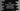# if-else condition in Dart explanation with example# if-else condition in dart :

if-else statements are control flow statements. Using these statements, you can control the flow of a dart program.

Basically, the if block comes before else. if block checks for a condition. If the condition is true it executes the code written in that block. Else, it executes the code written in the else block.

The syntax of dart if-else is similar to if-else of any other programming languages. In this tutorial, we will learn how to use if-else condition in dart with different examples.

## The syntax of if-else :

The syntax of dart if-else looks like as below :

``````if(condition){
// if-block
}else{
// else-block
}``````

It checks the condition first. If it is true, it will execute the code written in if-block. Else, it will execute the else-block.

Note that else is optional. The below syntax is also valid in dart :

``````if(condition){
// if-block
}``````

We don’t have any else statement here. It will only check the condition and execute the if-block if the condition is true.

### Example of if-else without else :

``````import 'dart:io';

main() {
print("Enter a number : ");

if (n > 50) {
print("\$n is greater than 50");
}

if (n % 5 == 0) {
print("\$n is divisible by 5");
}
}``````

### Explanation :

In the above example, we are only using if blocks without any else block. Two if blocks are here. The first one checks if the user input number is greater than 50 or not and the second if block checks if the number is divisible by 5 or not.

It prints one message if the condition in the if block is true.

The output may differ based on the input number. For example :

``````Enter a number :
60
60 is greater than 50
60 is divisible by 5

Enter a number :
45
45 is divisible by 5

Enter a number :
66
66 is greater than 50

Enter a number :
33``````

### Example of if-else with else :

Now, let’s try with if-else block :

``````main() {
print("Enter a number : ");

if (n > 50) {
print("\$n is greater than 50");
}else{
print("\$n is less than 50");
}
}``````

This example will take one user input number and first of all, it will check if it is greater than 50 or not. If it is greater than 50, it will print one message. Else, it will print the message of the else block i.e. the number is less than 50.

### if-else if-else block :

if else-if else block is similar to the if-else block. It’s syntax is :

``````if(condition){
// code block for if
}else if(condition-2){
// code block for else-if
}else{
// code block for else
}``````

We can add one or many else if block in between if and else. The conditions are checked from top to bottom. If any condition is true, it will execute that block.

``````import 'dart:io';

main() {
print("Enter a number : ");

if (n > 50) {
print("\$n is greater than 50");
} else if (n < 40 && n > 30) {
print("\$n is less than 40 and greater than 30");
} else if (n < 50 && n > 40) {
print("\$n is less than 50 and greater than 40");
} else {
print("\$n is less than 30");
}
}``````

In this example, we are using two else if blocks. if block is checked first, first else if is checked second, second else if is checked third and the last else is checked at last.

Example output :

``````Enter a number :
55
55 is greater than 50

Enter a number :
45
45 is less than 50 and greater than 40

Enter a number :
35
35 is less than 40 and greater than 30

Enter a number :
25
25 is less than 30``````